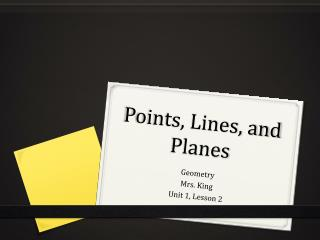DownloadDownload PresentationPoints, Lines, and Planes

# Points, Lines, and Planes

Télécharger la présentation## Points, Lines, and Planes

- - - - - - - - - - - - - - - - - - - - - - - - - - - E N D - - - - - - - - - - - - - - - - - - - - - - - - - - -
##### Presentation Transcript

1. Points, Lines, and Planes Geometry Mrs. King Unit 1, Lesson 2

2. A P Q Z Definition Point: a location in space. A point has no size, but is represented by a dot labeled with a capital letter.

3. Definition Space: the set of all points

4. l Q P Definition Line: a series of points that extends without end in two opposite directions.

5. Q R P Definition Collinear: points that lie on the same line.

6. In the figure below, name three points that are collinear and three points that are not collinear. Practice Points Y, Z, and W lie on a line, so they are collinear. For example, X, Y, and Z and X, W, and Z form triangles and are not collinear.

7. Definition Plane: a flat surface that extends in all directions without end.

8. Practice Name the plane shown in two different ways. You can name a plane using any three or more points on that plane that are not collinear. Some possible names for the plane shown are: plane RST plane RSU plane RTU plane STU plane RSTU

9. Definition Coplanar: points and lines that are in the same plane.

10. Practice • How many planes are represented by the surfaces of the cube? • Name the plane of the front of the cube in two different ways. • Name a point that is coplanar with the given points: • E, F, G • B, C, G

11. Definition Postulate: an accepted statement of fact.

12. Four Basic Postulates 1-1: Through any two points there is exactly one line. 1-2: If two lines intersect, then they intersect in exactly one point. 1-3: If two planes intersect, then they intersect in a line. 1-4: Through any three noncollinear points there is exactly one plane.

13. Homework Points, Lines, and Planes in Student Practice Packet (Page 3, #1-21)